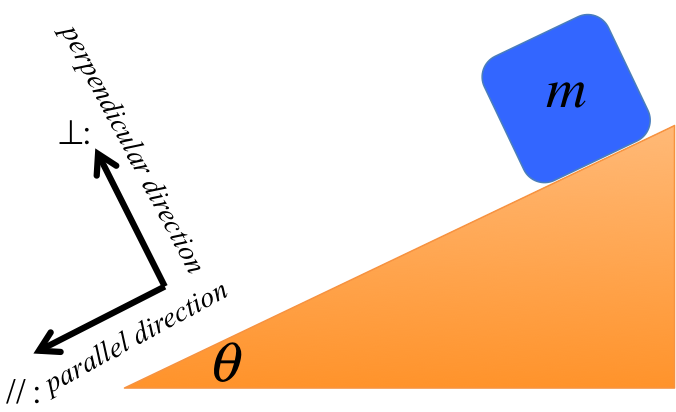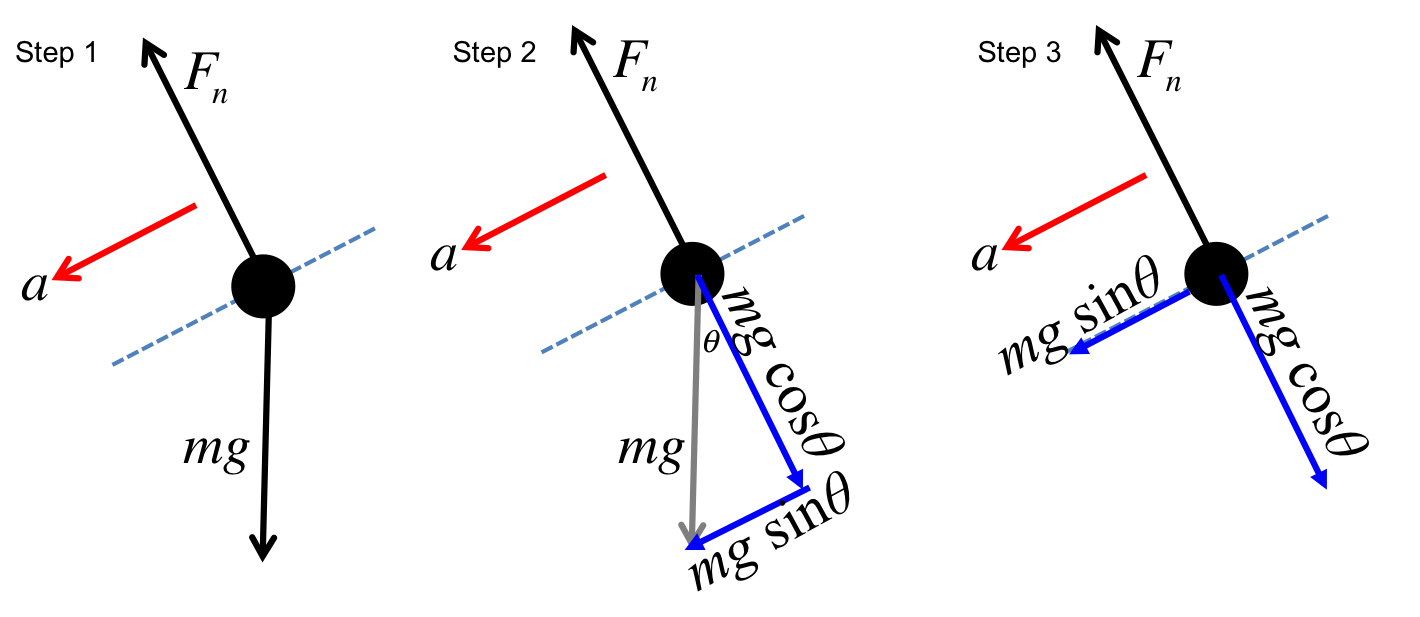# PHYS 170

Lecture 04b - Newton's Laws

## Introduction

In this lecture we will study two main concepts:

• Object on an incline
• Force on multiple objects
To analyse how an object slides down an incline we will have to decompose the forces carefully into the parallel and perpendicular directions. As for multi-body problems,the key idea is "divide and conquer". By considering the force diagram of each object independently, one can break a complicated multi-body problem into multiple simpler single-body problems.

## Object on A Frictionless Incline

### Simulation - frictionless incline (click to hide)

Canvas not supported
Drag on the surface of the incline to change the angle.
The force diagram can also be dragged to a different location.
The length of the track is $20m$. The mass of the block is $1kg$.

There are only two forces on an object sliding down an incline (see simulation above):

• Force of gravity $F_g = mg$
• Normal force $F_n$
The main trick to solving this problem is to decompose the forces into the parallel and the perpendicular directions, as seen in the figure below.Along the parallel direction, there is only one force left ($mg\sin\theta$). The 2nd Law then gives: $$\begin{eqnarray} mg\sin\theta&=& ma \\ \Rightarrow a &=& g\sin\theta \end{eqnarray}$$

Along the perpendicular direction, there is no acceleration (i.e. $a_\perp = 0$), implying the two forces ($F_n$ and $mg\cos\theta$) in the perpendicular direction cancel out: $$\begin{eqnarray} F_n&=& mg\cos\theta \end{eqnarray}$$